Home Practice
For learners and parents For teachers and schools
Textbooks
Mathematics
JSS 1 JSS 2 JSS 3
Full catalogue
Pricing SupportLog in

We think you are located in Nigeria. Is this correct?

# Test yourself now

High marks in science are the key to your success and future plans. Test yourself and learn more on Siyavula Practice.

# Chapter 6: Simultaneous linear equations

In Chapter 5 you learnt that we solve an equation when we find the value of the variable that makes the equation true. This is called the solution of the equation.

Simultaneous equations are two or more equations for which at least one value of the variables make both equations true. We say simultaneous equations are satisfied by the same set of values.

The following two equations, for example, are both satisfied if $x=2$:

simultaneous equations Simultaneous equations are two or more equations that are satisfied by the same set of values for the variables.

Last year you learnt that an algebraic equation can give the relationship between the $x$-coordinates and the $y$-coordinates of a collection of points. Here are some of the equations you worked with:

You learnt how to plot points to form a pattern or shape called a graph. When the graph plotted for an equation forms a straight line, we say the equation is a linear equation.

graph A graph is a pattern formed by points whose coordinates are obtained from a given equation.

linear equation A linear equation is an equation between two variables that gives a straight line when plotted on a graph.

In this chapter you will learn different methods to solve simultaneous linear equations.

### 6.1 Tables and graphs

The following worked example revises how to plot a graph.

### Worked example 6.1: Plotting a graph

Plot a graph for the equation $y=3x-1$.

1. Step 1: Compile a table of values.

• Choose at least five $x$-values.
• Replace the $x$ in the equation with each of the values you chose.
• Calculate the corresponding $y$-values.
\begin{array}{|c|c|c|c|c|c|} \hline x & -2 & -1 & \;0\; & \;1\; & \;2\; \\ \hline y & -7 & -4 & -1 & 2 & 5 \\ \hline \end{array}
2. Step 2: Using the correct format, write down the coordinates of the points represented in the table of values.

3. Step 3: Draw the axes of the Cartesian plane correctly.

• The axes must be perpendicular to each other.
• There must be an arrowhead at both ends of each axis.
• Label the horizontal axis $x$ and the vertical axis $y$.
• Label the origin with the number $0$.
• Insert equal divisions on each axis. Label them with whole numbers, as you would do for a number line.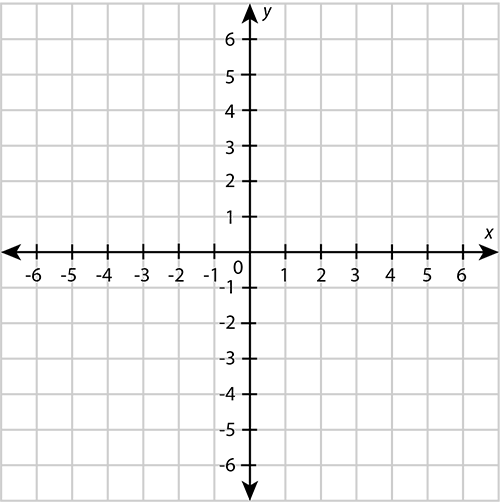4. Step 4: Plot each of the points from Step 2.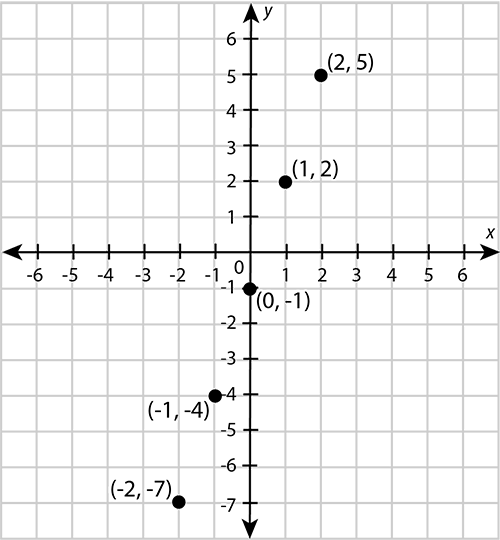5. Step 5: Draw a line through the points to get the graph.

These points form a straight line, so you must use a ruler to draw the line.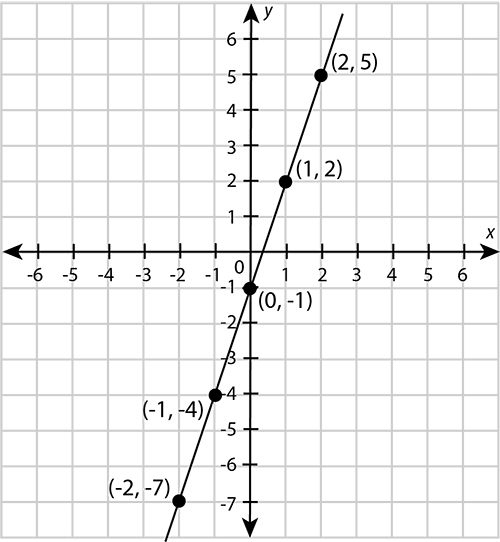We can solve simultaneous linear equations by plotting graphs for the equations on the same set of axes. The following worked example shows how this is done.

### Worked example 6.2: Using tables of values and graphs to solve simultaneous linear equations

Use graphs to solve the following simultaneous equations:

1. Step 1: Compile a table of values for each equation.

For $y=-4x+3$:

\begin{array}{|c|c|c|c|c|c|} \hline x & -2 & -1 & \;0\; & \;1\; & \;2\; \\ \hline y & 11 & 7 & 3 & -1 & -5 \\ \hline \end{array}

It is easier to find the values of $y$ for your values of $x$ if you first write the equation so that it has only $y$ on the left-hand side of the equals sign.

\begin{align} 3y & = x-4 \\ y & = \frac{x-4}{3} \end{align}

For $y=\frac{x-4}{3}$:

\begin{array}{|c|c|c|c|c|c|} \hline x & -2 & -1 & \;0\; & \;1\; & \;2\; \\ \hline y & -2 & -\frac{5}{3} & -\frac{4}{3} & -1 & -\frac{2}{3} \\ \hline \end{array}
2. Step 2: Using the correct format, write down the coordinates of the points represented in the table of values.

For $y=-4x+3$:

For $y=\frac{x-4}{3}$:

3. Step 3: Draw the axes of the Cartesian plane correctly and plot the first graph.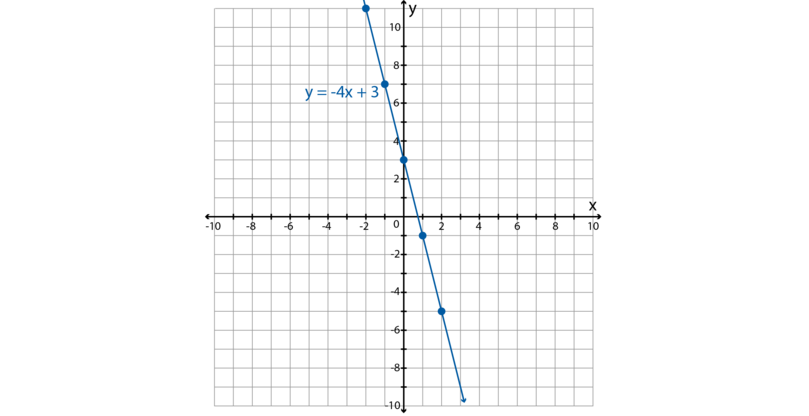4. Step 4: On the same set of axes you used in Step 3, plot the second graph.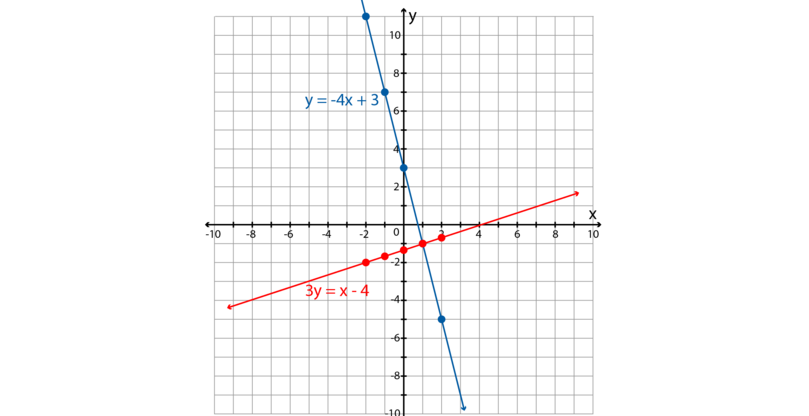5. Step 5: Determine the coordinates of the point at which the two graphs intersect.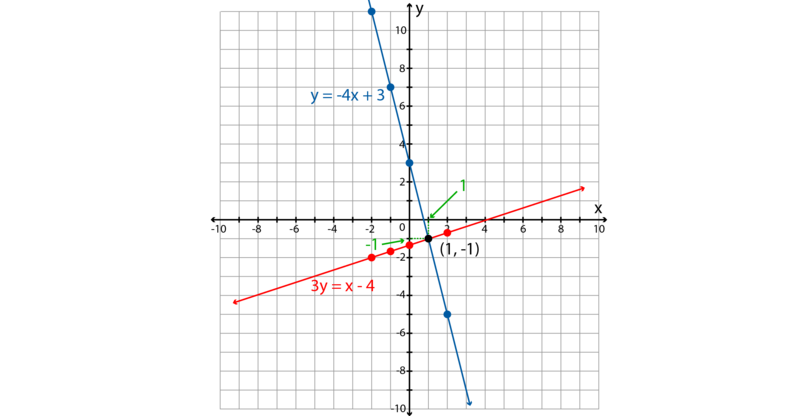From the graph, we can see that the coordinates of the point where the two lines cross are $(1, -1)$.

6. Step 6: Compare your answer in Step 5 with the tables of values in Step 1.

Both tables have the values $y=-1$ for $x=1$.

This means the solution is $(1, -1)$.

7. Step 7: Check your solution. Remember to substitute the solutions for the variables into the original equations.

\begin{align} y&=-4x+3 \\ -1&=-4(1)+3\\ -1&=-4+3\\ -1&=-1\\ \therefore \text{LHS}&=\text{RHS} \end{align} \begin{align} 3y&=x-4 \\ 3(-1)&=1-4\\ -3&=-3\\ \therefore \text{LHS}&=\text{RHS} \end{align}

The solution $(1, -1)$ satisfies both equations.

### Exercise 6.1: Use tables of values and graphs to solve simultaneous linear equations

Use graphs to solve the each of the following pairs of simultaneous equations.

1. $y=3x+2$ and $y=x+4$

\begin{array}{|c|c|c|c|c|c|} \hline x & -2 & -1 & \;0\; & \;1\; & \;2\; \\ \hline y & -4 & -1 & 2 & 5 & 8 \\ \hline \end{array} \begin{array}{|c|c|c|c|c|c|} \hline x & -2 & -1 & \;0\; & \;1\; & \;2\; \\ \hline y & 2 & 3 & 4 & 5 & 6 \\ \hline \end{array}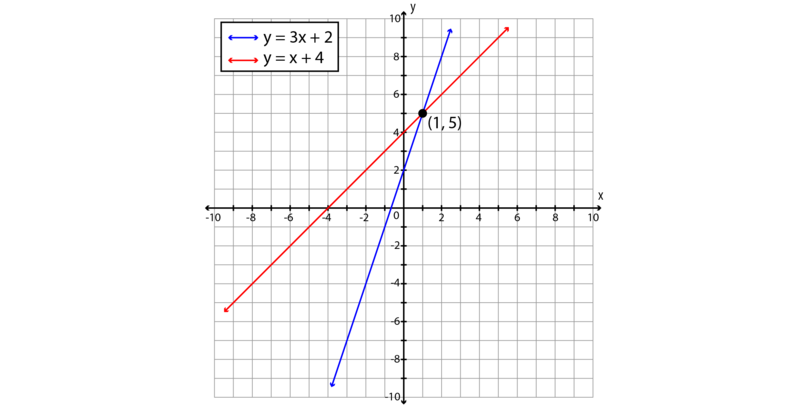The solution is $(1, 5)$.

2. $2y=x-2$ and $4y=-5x-4$

\begin{array}{|c|c|c|c|c|c|} \hline x & -2 & -1 & \;0\; & \;1\; & \;2\; \\ \hline y & -2 & -1\frac{1}{2} & -1 & -\frac{1}{2} & 0 \\ \hline \end{array} \begin{array}{|c|c|c|c|c|c|} \hline x & -2 & -1 & \;0\; & \;1\; & \;2\; \\ \hline y & 1\frac{1}{2} & \frac{1}{4} & -1 & -2\frac{1}{4} & -3\frac{1}{2} \\ \hline \end{array}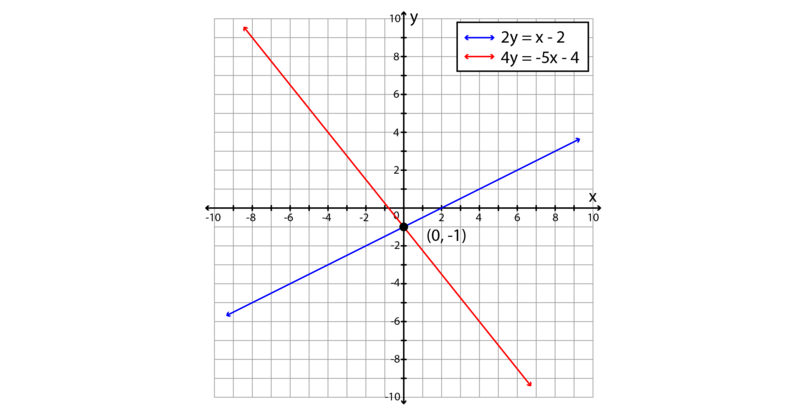The solution is $(0, -1)$.

3. $5y=-5x-15$ and $5y=x-3$

\begin{array}{|c|c|c|c|c|c|} \hline x & -2 & -1 & \;0\; & \;1\; & \;2\; \\ \hline y & -1 & -2 & -3 & -4 & -5 \\ \hline \end{array} \begin{array}{|c|c|c|c|c|c|} \hline x & -2 & -1 & \;0\; & \;1\; & \;2\; \\ \hline y & -1 & -\frac{4}{5} & -\frac{3}{5} & -\frac{2}{5} & -\frac{1}{5} \\ \hline \end{array}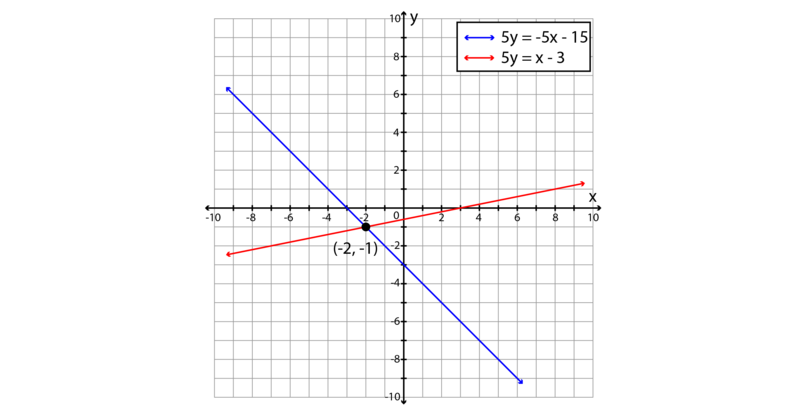The solution is $(-2, -1)$.

4. $y=-\frac{3x}{2}+5$ and $y=2x-2$

\begin{array}{|c|c|c|c|c|c|} \hline x & -2 & -1 & \;0\; & \;1\; & \;2\; \\ \hline y & 8 & 6\frac{1}{2} & 5 & 3\frac{1}{2} & 2 \\ \hline \end{array} \begin{array}{|c|c|c|c|c|c|} \hline x & -2 & -1 & \;0\; & \;1\; & \;2\; \\ \hline y & -6 & -4 & -2 & 0 & 2 \\ \hline \end{array}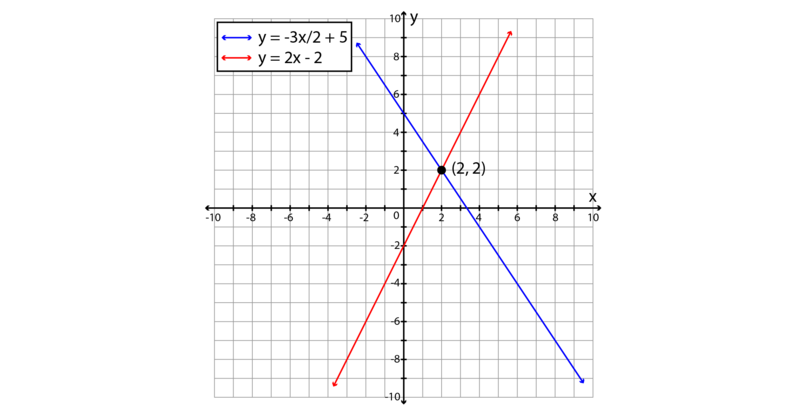The solution is $(2, 2)$.

5. $y=-5x-4$ and $4y=x+5$

\begin{array}{|c|c|c|c|c|c|} \hline x & -2 & -1 & \;0\; & \;\frac{1}{2}\; & \;1\; \\ \hline y & 6 & 1 & -4 & -6\frac{1}{2} & -9 \\ \hline \end{array}

If $x=2$, then $y=-14$, which does not fit on our set of axes. In such a case, choose another $x$-value that does fit on the axes, such as $\frac{1}{2}$.

\begin{array}{|c|c|c|c|c|c|} \hline x & -2 & -1 & \;0\; & \;1\; & \;2\; \\ \hline y & \frac{3}{4} & 1 & 1\frac{1}{4} & 1\frac{1}{2} & 1\frac{3}{4} \\ \hline \end{array}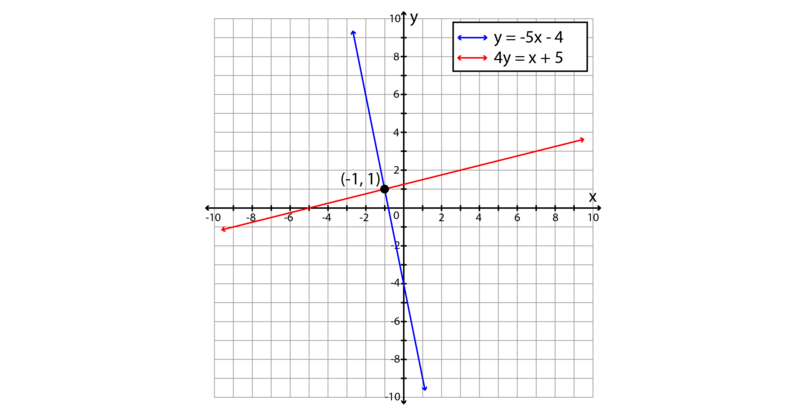The solution is $(-1, 1)$.

6. $2y=4x-4$ and $5y=4x+14$

\begin{array}{|c|c|c|c|c|c|} \hline x & -2 & -1 & \;0\; & \;1\; & \;2\; \\ \hline y & -6 & -4 & -2 & 0 & 2 \\ \hline \end{array} \begin{array}{|c|c|c|c|c|c|} \hline x & -2 & -1 & \;0\; & \;1\; & \;2\; \\ \hline y & 1\frac{1}{5} & 2 & 2\frac{4}{5} & 3\frac{3}{5} & 4\frac{2}{5} \\ \hline \end{array}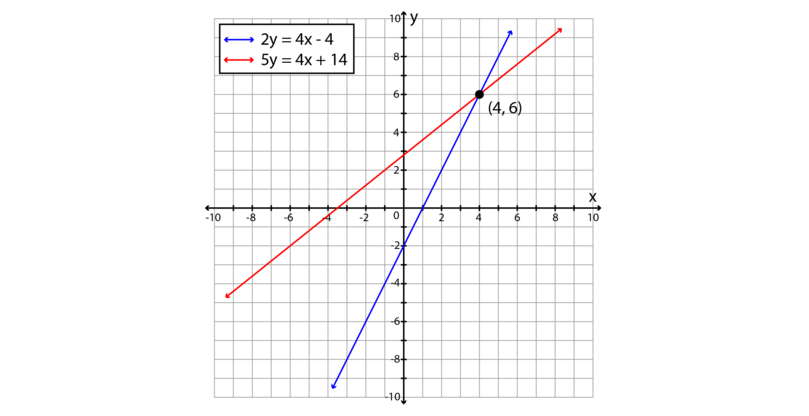The solution is $(4, 6)$.

7. $2x+2=2y$ and $5x-11=3y$

Remember that it will help you to draw up the tables of values if you rewrite the equation to have only $y$ on the left-hand side of the equals sign.

\begin{align} 2x+2 &=2y \\ 2y &=2x+2 \\ y &=\frac{2x+2}{2} \\ \end{align} \begin{array}{|c|c|c|c|c|c|} \hline x & -2 & -1 & \;0\; & \;1\; & \;2\; \\ \hline y & -1 & 0 & 1 & 2 & 3 \\ \hline \end{array} \begin{align} 5x-11 &=3y \\ 3y &=5x-11 \\ y &=\frac{5x-11}{3} \\ \end{align} \begin{array}{|c|c|c|c|c|c|} \hline x & -2 & -1 & \;0\; & \;1\; & \;2\; \\ \hline y & -7 & -5\frac{1}{3} & -3\frac{2}{3} & -2 & -\frac{1}{3} \\ \hline \end{array}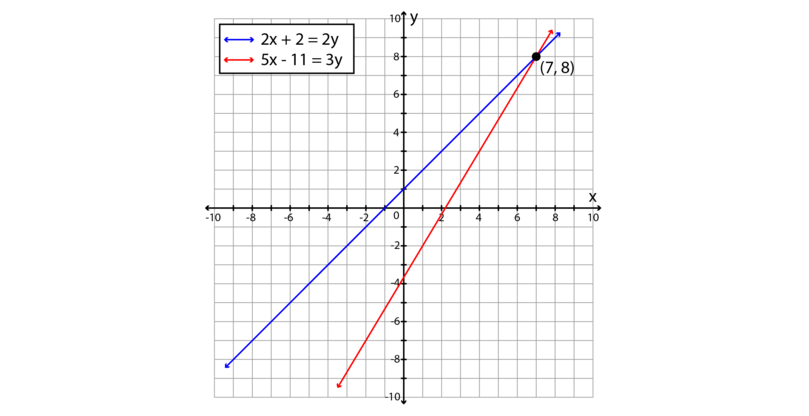The solution is $(7, 8)$.

8. $5x+4=-4y$ and $-4x+4=4y$

\begin{align} 5x+4 &=-4y \\ -4y &=5x+4 \\ y &=-\frac{5x+4}{4} \\ \end{align} \begin{array}{|c|c|c|c|c|c|} \hline x & -2 & -1 & \;0\; & \;1\; & \;2\; \\ \hline y & 1\frac{1}{2} & \frac{1}{4} & -1 & -2\frac{1}{4} & -3\frac{1}{2} \\ \hline \end{array} \begin{align} -4x+4 &=4y \\ 4y &=-4x+4 \\ y &=\frac{-4x+4}{4} \\ \end{align} \begin{array}{|c|c|c|c|c|c|} \hline x & -2 & -1 & \;0\; & \;1\; & \;2\; \\ \hline y & 3 & 2 & 1 & 0 & -1 \\ \hline \end{array}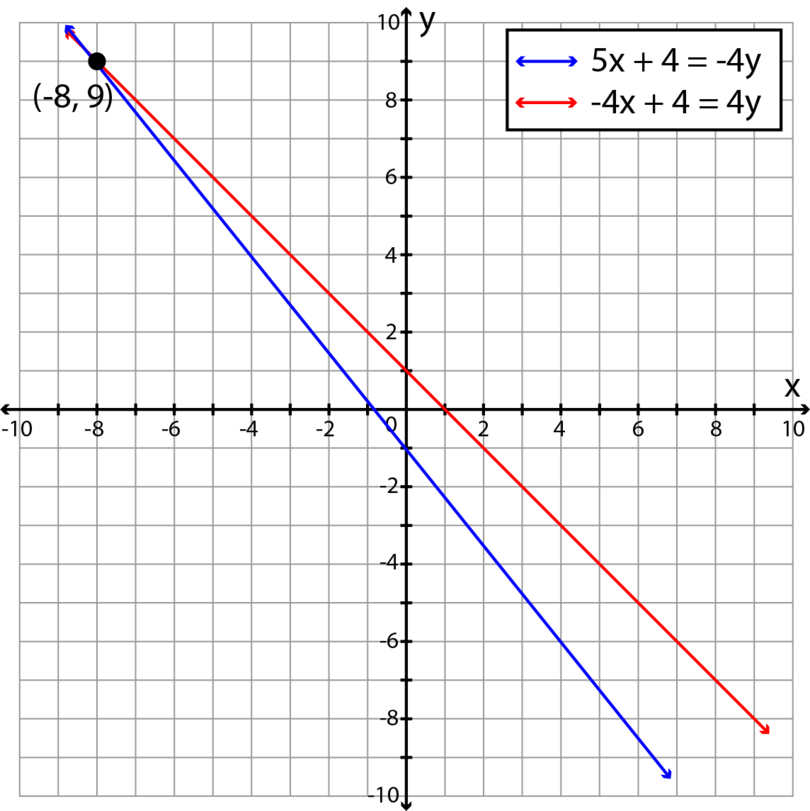The solution is $(-8, 9)$.

9. $3x+5y=-19$ and $-3x+4y=1$

\begin{align} 3x+5y &=-19 \\ 5y &=-3x-19 \\ y &=\frac{-3x-19}{5} \\ \end{align} \begin{array}{|c|c|c|c|c|c|} \hline x & -2 & -1 & \;0\; & \;1\; & \;2\; \\ \hline y & -2\frac{3}{5} & -3\frac{1}{5} & -3\frac{4}{5} & -4\frac{2}{5} & -5 \\ \hline \end{array} \begin{align} -3x+4y &=1 \\ 4y &=3x+1 \\ y &=\frac{3x+1}{4} \\ \end{align} \begin{array}{|c|c|c|c|c|c|} \hline x & -2 & -1 & \;0\; & \;1\; & \;2\; \\ \hline y & -1\frac{1}{4} & -\frac{1}{2} & \frac{1}{4} & 1 & 1\frac{3}{4} \\ \hline \end{array}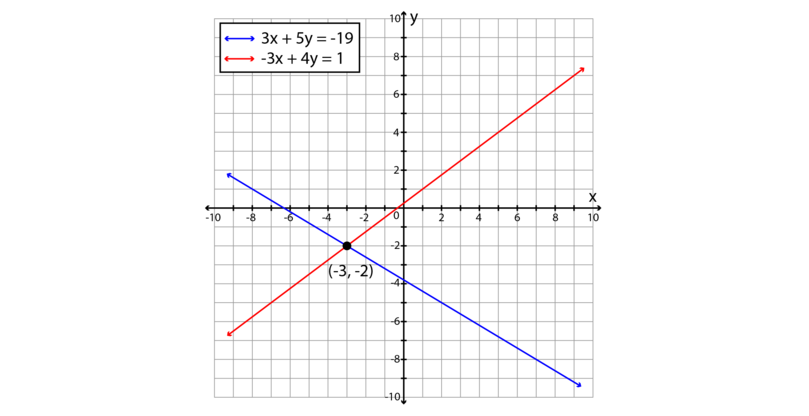The solution is $(-3, -2)$.

10. $2x-4y=-14$ and $-2x+3y=13$

\begin{align} 2x-4y &=-14 \\ -4y &=-2x-14 \\ y &=\frac{-2x-14}{-4} \\ y &=\frac{2x+14}{4} \\ \end{align} \begin{array}{|c|c|c|c|c|c|} \hline x & -2 & -1 & \;0\; & \;1\; & \;2\; \\ \hline y & 2\frac{1}{2} & 3 & 3\frac{1}{2} & 4 & 4\frac{1}{2} \\ \hline \end{array} \begin{align} -2x+3y &=13 \\ 3y &=2x+13 \\ y &=\frac{2x+13}{3} \\ \end{align} \begin{array}{|c|c|c|c|c|c|} \hline x & -2 & -1 & \;0\; & \;1\; & \;2\; \\ \hline y & 3 & 3\frac{2}{3} & 4\frac{1}{3} & 5 & 5\frac{2}{3} \\ \hline \end{array}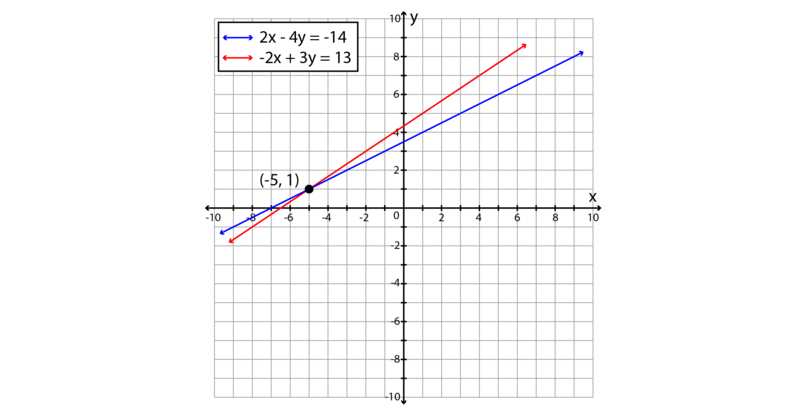The solution is $(-5, 1)$.

## 6.2 Elimination method

The elimination method is another method you can use to solve simultaneous linear equations. The following worked example shows how it works.

### Worked example 6.3: Using the elimination method to solve simultaneous linear equations

Use the elimination method to solve the following simultaneous equations:

1. Step 1: Write one equation below the other. Line up the terms that have the same variables and line up the constant terms. Label one equation (1) and the other equation (2).

\begin{array}{r r r r r r} 3x & + & 4y & = & 25 & \dots (1) \\ 2x & - & y & = & 2 & \dots (2) \\ \end{array}
2. Step 2: Multiply one or both equations so that you get two lined-up terms that have the same numeric coefficient.

The aim of this method is to eliminate (remove) one of the variables. We do this by adding or subtracting lined-up terms so that the answer for one of the variables is zero. For example, $4y+(-4y)=0$.

\begin{array}{l r r r r r r} (1): & 3x & + & 4y & = & 25 & \dots (1) \\ (2) \times 4: & \color{red}8\color{black}x & - & \color{red}{4}\color{black}y & = & \color{red}8 & \dots (3) \\ \end{array}

Note that every term in equation (2) is multiplied by $4$, which means that the value of the equation stays the same. Number the new equation as (3).

3. Step 3: Add or subtract the equations from Step 2 so that one lined-up pair gives zero as an answer.

In this case we add to eliminate the $y$ term, so we add the other terms also.

\begin{array}{l r r r r r r} (1): & 3x & + & 4y & = & 25 & \dots (1) \\ (2) \times 4: & \color{red}8\color{black}x & - & \color{red}{4}\color{black}y & = & \color{red}8 & \dots (3) \\ \hline (1)+(3): & 11x & & & = & 33 & \end{array}
4. Step 4: Solve the equation obtained in Step 3.

\begin{align} 11x&=33\\ \frac{11x}{11}&=\frac{33}{11} \\ \therefore x&=3 \end{align}
5. Step 5: Substitute the answer from Step 4 into one of the original equations and solve.

We need to get both the $x$ and $y$ values to solve these simultaneous equations. We have the $x$ value, so to get the $y$ value, we can substitute into either of the equations. Both equations will give the same value for $y$.

Substitute $x=3$ in equation (2):

\begin{align} 2x-y&=2\\ 2(3)-y&=2 \\ 6-6-y&=2-6 \\ -y&=-4\\ \frac{-y}{-1}&=\frac{-4}{-1} \\ \therefore y&=4 \end{align}
6. Step 6: Check your solution. Remember to replace the variables in the original equations.

For $x=3$ and $y=4$:

\begin{align} 3x+4y&=25 \\ 3(3)+4(4)&=25\\ 9+16&=25\\ 25&=25\\ \therefore \text{LHS}&=\text{RHS} \end{align} \begin{align} 2x-y&=2 \\ 2(3)-4&=2\\ 6-4&=2\\ 2&=2\\ \therefore \text{LHS}&=\text{RHS} \end{align}

The solution $x=3$ and $y=4$ satisfies both equations.

You can also write the solution in coordinate form: $(3, 4)$.

### Exercise 6.2: Use the elimination method to solve simultaneous linear equations

Use the elimination method to solve the each of the following pairs of simultaneous equations.

1. $x-3y=5$ and $-5x+3y=11$

\begin{array}{l r r r r r r} & x & - & 3y & = & 5 & \dots (1) \\ & -5x & + & 3y & = & 11 & \dots (2) \\ \hline (1)+(2): & -4x & & & = & 16 & \end{array} \begin{align} -4x &= 16 \\ \therefore x & =-4 \end{align}

Substitute in (1):

\begin{align} x-3y &= 5 \\ -4 -3y &= 5 \\ -3y &= 9 \\ \therefore y &= -3 \end{align}

Answer: $x = -4$ and $y = -3$

2. $x+y=6$ and $y-x=2$

\begin{array}{l r r r r r r} & x & + & y & = & 6 & \dots (1) \\ & -x & + & y & = & 2 & \dots (2) \\ \hline (1)-(2): & 2x & & & = & 4 & \end{array}

Remember that $x-(-x)=x+x=2x$.

\begin{align} 2x &= 4 \\ \therefore x & =2 \end{align}

Substitute in (1):

\begin{align} x+y &= 6 \\ 2+y &= 6 \\ y &= 6-2 \\ \therefore y &= 4 \end{align}

Answer: $x = 2$ and $y = 4$

3. $2x+5y=12$ and $y=-12-2x$

\begin{align} y&=-12-2x \\ y+2x&=-12-2x+2x\\ \therefore y+2x&=-12 \end{align} \begin{array}{l r r r r r r} & 2x & + & 5y & = & 12 & \dots (1) \\ & 2x & + & y & = & -12 & \dots (2) \\ \hline (1)-(2): & & & 4y & = & 24 & \end{array} \begin{align} 4y &= 24 \\ \therefore y & =6 \end{align}

Substitute in (1):

\begin{align} 2x+5y &= 12 \\ 2x +5(6) &= 12 \\ 2x &= 12-30 \\ 2x &= -18 \\ \therefore x &= -9 \end{align}

Answer: $x = -9$ and $y = 6$

4. $x-3y=11$ and $4x+2y=30$

\begin{array}{l r r r r r r} & x & - & 3y & = & 11 & \dots (1) \\ & 4x & + & 2y & = & 30 & \dots (2) \\ \hline (1)\times 4: & 4x & - & 12y & = & 44 & \dots (3) \\ (2): & 4x & + & 2y & = & 30 & \dots (2) \\ \hline (3)-(2): & & & -14y & = & 14 & \end{array} \begin{align} -14y &= 14 \\ \therefore y & =-1 \end{align}

Substitute in (1):

\begin{align} x-3y&=11 \\ x -3(-1) &= 11 \\ x +3 &= 11 \\ x &= 11-3 \\ \therefore x &= 8 \end{align}

Answer: $x = 8$ and $y = -1$

5. $-6x+4y=-20$ and $3x+y=-5$

\begin{array}{l r r r r r r} & -6x & + & 4y & = & -20 & \dots (1) \\ & 3x & + & y & = & -5 & \dots (2) \\ \hline (1): & -6x & + & 4y & = & -20 & \dots (1) \\ (2)\times 4: & 12x & + & 4y & = & -20 & \dots (3) \\ \hline (1)-(3): & -18x & & & = & 0 & \end{array} \begin{align} -18x &= 0 \\ \therefore x & =0 \end{align}

Substitute in (2):

\begin{align} 3x+y&=-5 \\ 3(0)+y &= -5 \\ \therefore y &= -5 \end{align}

Answer: $x = 0$ and $y = -5$

6. $2x+3y=-2$ and $6x+5y=2$

\begin{array}{l r r r r r r} & 2x & + & 3y & = & -2 & \dots (1) \\ & 6x & + & 5y & = & 2 & \dots (2) \\ \hline (1)\times 3: & 6x & + & 9y & = & -6 & \dots (3) \\ (2): & 6x & + & 5y & = & 2 & \dots (2) \\ \hline (3)-(2): & & & 4y & = & -8 & \end{array} \begin{align} 4y &= -8 \\ \therefore y & = -2 \end{align}

Substitute in (1):

\begin{align} 2x+3y&=-2 \\ 2x+3(-2) &= -2 \\ 2x-6&=-2\\ 2x&=4\\ \therefore x &= 2 \end{align}

Answer: $x = 2$ and $y = -2$

7. $3y-6x=-36$ and $y-5x=-27$

\begin{array}{l r r r r r r} & 3y & - & 6x & = & -36 & \dots (1) \\ & y & - & 5x & = & -27 & \dots (2) \\ \hline (1): & 3y & - & 6x & = & -36 & \dots (1) \\ (2)\times 3: & 3y & - & 15x & = & -81 & \dots (3) \\ \hline (1)-(3): & & & 9x & = & 45 & \end{array} \begin{align} 9x &= 45 \\ \therefore x & = 5 \end{align}

Substitute in (2):

\begin{align} y-5x&=-27 \\ y-5(5)&=-27 \\ y-25&=-27\\ y&=-27+25\\ \therefore y &= -2 \end{align}

Answer: $x = 5$ and $y = -2$

8. $y=-3x-23$ and $-2x=-14-3y$

\begin{array}{l r r r r r r} & y & + & 3x & = & -23 & \dots (1) \\ & 3y & - & 2x & = & -14 & \dots (2) \\ \hline (1)\times 3: & 3y & + & 9x & = & -69 & \dots (3) \\ (2): & 3y & - & 2x & = & -14 & \dots (2) \\ \hline (3)-(2): & & & 11x & = & -55 & \end{array} \begin{align} 11x &= -55 \\ \therefore x & = -5 \end{align}

Substitute in (1):

\begin{align} y&=-3x-23 \\ y&=-3(-5)-23 \\ y&=15-23\\ \therefore y &= -8 \end{align}

Answer: $x = -5$ and $y = -8$

9. $4x+3y=1$ and $5x+2y=3$

If it is not obvious by what number to multiply, find the LCM (lowest common multiple) of two lined-up terms. Then multiply both equations so that you get the LCM. For example, the LCM of 4 and 5 is 20.

\begin{array}{l r r r r r r} & 4x & + & 3y & = & 1 & \dots (1) \\ & 5x & + & 2y & = & 3 & \dots (2) \\ \hline (1)\times 5: & 20x & + & 15y & = & 5 & \dots (3) \\ (2)\times 4: & 20x & + & 8y & = & 12 & \dots (4) \\ \hline (3)-(4): & & & 7y & = & -7 & \end{array} \begin{align} 7y &= -7 \\ \therefore y & = -1 \end{align}

Substitute in (2):

\begin{align} 5x+2y&=3 \\ 5x+2(-1)&=3 \\ 5x-2&=3\\ 5x&=5\\ \therefore x &= 1 \end{align}

Answer: $x = 1$ and $y = -1$

10. $7x=13+3y$ and $2y=-4x$

\begin{align} 2y&=-4x \\ 2y+4x&=-4x+4x\\ 2y+4x&=0 \end{align} \begin{array}{l r r r r r r} & 7x & - & 3y & = & 13 & \dots (1) \\ & 4x & + & 2y & = & 0 & \dots (2) \\ \hline (1)\times 2: & 14x & - & 6y & = & 26 & \dots (3) \\ (2)\times 3: & 12x & + & 6y & = & 0 & \dots (4) \\ \hline (3)+(4): & 26x & & & = & 26 & \end{array} \begin{align} 26x &= 26 \\ \therefore x & = 1 \end{align}

Substitute in (2):

\begin{align} 4x+2y&=0 \\ 4(1)+2y&=0 \\ 2y&=-4\\ \therefore y &= -2 \end{align}

Answer: $x = 1$ and $y = -2$

## 6.3 Substitution method

The substitution method is a third method you can use to solve simultaneous linear equations. The following worked example shows how it works.

### Worked example 6.4: Using the substitution method to solve simultaneous linear equations

Use the substitution method to solve the following simultaneous equations:

1. Step 1: For one of the equations, make one of the variables the subject of the equation. Label this equation (1).

To make a variable the subject of an equation, rearrange the equation so that the variable is on the left-hand side of the equals sign with 1 as its coefficient.

\begin{align} 3x+y&=8 \\ 3x-3x+y&=8-3x \\ \therefore y&=-3x+8 \phantom{00}\dots (1) \end{align}

It does not matter which equation you choose for the first step. The rest of the method will be easier if you choose the equation that will not give you fractions when you rearrange it.

2. Step 2: Label the remaining equation (2). Substitute the value for $y$ from equation (1) for $y$ in equation (2).

In the place of the variable $y$ in equation (2), put the expression given on the right-hand side of the equals sign in equation (1).

Substitute (1) in (2):

\begin{align} 4x-3y&=2 \\ 4x-3(-3x+8)&=2 \end{align}
3. Step 3: Expand the brackets and solve the equation.

\begin{align} 4x-3y&=2 \\ 4x-3(-3x+8)&=2\\ 4x+9x-24&=2\\ 13x&=2+24\\ 13x&=26\\ \therefore x&=2 \end{align}
4. Step 4: Substitute the answer from Step 3 into equation (1) and solve.

\begin{align} y&=-3x+8\\ &=-3(2)+8 \\ &=-6+8 \\ &=2\\ \end{align}
5. Step 6: Check your solution. Remember to replace the variables in the original equations.

For $x=2$ and $y=2$:

\begin{align} 3x+y&=8 \\ 3(2)+(2)&=8\\ 6+2&=8\\ 8&=8\\ \therefore \text{LHS}&=\text{RHS} \end{align} \begin{align} 4x-3y&=2 \\ 4(2)-3(2)&=2\\ 8-6&=2\\ 2&=2\\ \therefore \text{LHS}&=\text{RHS} \end{align}

The solution $x=2$ and $y=2$ satisfies both equations.

You can also write the solution in coordinate form: $(2, 2)$.

### Exercise 6.3: Use the substitution method to solve simultaneous linear equations

Use the substitution method to solve the each of the following pairs of simultaneous equations.

1. $y-3x=0$ and $2y+5x=11$

\begin{align} y-3x&=0\\ y&=3x\phantom{00} \dots (1) \end{align}

And:

Substitute (1) in (2):

\begin{align} 2(3x)+5x&=11 \\ 6x+5x&=11 \\ 11x&=11 \\ \therefore x &=1 \end{align}

Substitute in (1):

\begin{align} y&=3(1) \\ &= 3 \end{align}

Answer: $x = 1$ and $y = 3$

2. $y+x=7$ and $2x-2y=6$

\begin{align} y+x&=7\\ y&=-x+7\phantom{00} \dots (1) \end{align}

And:

Substitute (1) in (2):

\begin{align} 2x-2(-x+7)&=6 \\ 2x+2x-14&=6 \\ 4x&=20 \\ \therefore x &=5 \end{align}

Substitute in (1):

\begin{align} y&=-5+7 \\ &= 2 \end{align}

Answer: $x = 5$ and $y = 2$

3. $y-3x=4$ and $5y-6x=2$

\begin{align} y-3x&=4\\ y&=3x+4\phantom{00} \dots (1) \end{align}

And:

Substitute (1) in (2):

\begin{align} 5(3x+4)-6x&=2 \\ 15x+20-6x&=2 \\ 9x&=2-20 \\ 9x&=-18x\\ \therefore x &=-2 \end{align}

Substitute in (1):

\begin{align} y&=3(-2)+4 \\ &= -6+4\\ &=-2 \end{align}

Answer: $x = -2$ and $y = -2$

4. $x-4y=3$ and $2x+y=15$

\begin{align} x-4y&=3\\ x&=3+4y\phantom{00} \dots (1) \end{align}

And:

Substitute (1) in (2):

\begin{align} 2(3+4y)+y&=15 \\ 6+8y+y&=15 \\ 9y&=15-6 \\ 9y&=9\\ \therefore y &=1 \end{align}

Substitute in (1):

\begin{align} x&=3+4(1) \\ &= 3+4\\ &=7 \end{align}

Answer: $x = 7$ and $y = 1$

5. $7y-x=1$ and $13y-2x=1$

\begin{align} 7y-x&=1\\ -x&=1-7y \\ x&=-1+7y\phantom{00} \dots (1) \end{align}

And:

Substitute (1) in (2):

\begin{align} 13y-2(-1+7y)&=1 \\ 13y+2-14y&=1 \\ -y&=1-2 \\ -y&=-1\\ \therefore y &=1 \end{align}

Substitute in (1):

\begin{align} x&=-1+7(1) \\ &= -1+7\\ &=6 \end{align}

Answer: $x = 6$ and $y = 1$

6. $1-3x=-7y$ and $2x+y=12$

\begin{align} 2x+y&=12\\ y&=12-2x \phantom{00} \dots (1) \end{align}

And:

Substitute (1) in (2):

\begin{align} 1-3x&=-7(12-2x) \\ 1-3x&=-84+14x \\ -3x-14x&=-84-1 \\ -17x&=-85\\ \therefore x &=5 \end{align}

Substitute in (1):

\begin{align} y&=12-2(5) \\ &= 12-10\\ &=2 \end{align}

Answer: $x = 5$ and $y = 2$

7. $2x+2y=12$ and $3y-3x=6$

\begin{align} 2x+2y&=12\\ 2x&=12-2y \\ x&=6-y\phantom{00} \dots (1) \end{align}

And:

Substitute (1) in (2):

\begin{align} 3y-3(6-y)&=6 \\ 3y-18+3y&=6 \\ 6y&=6+18 \\ 6y&=24\\ \therefore y &=4 \end{align}

Substitute in (1):

\begin{align} x&=6-4 \\ &=2 \end{align}

Answer: $x = 2$ and $y = 4$

8. $9=3x-3y$ and $2x+3y=4$

\begin{align} 9&=3x-3y\\ 3y&=3x-9 \\ y&=x-3\phantom{00} \dots (1) \end{align}

And:

Substitute (1) in (2):

\begin{align} 2x+3(x-3)&=4 \\ 2x+3x-9&=4 \\ 5x&=4+9 \\ 5x&=13\\ \therefore x &=\frac{13}{5} \end{align}

Substitute in (1):

\begin{align} y&=\frac{13}{5}-3 \\ &=\frac{13}{5}-\frac{15}{5}\\ &=-\frac{2}{5} \end{align}

Answer: $x = \frac{13}{5}$ and $y = -\frac{2}{5}$

9. $2x-3y=4$ and $20+5y=6x$

\begin{align} 2x-3y&=4\\ 2x&=3y+4 \\ x&=\frac{3}{2}y+2\phantom{00} \dots (1) \end{align}

And:

Substitute (1) in (2):

\begin{align} 20+5y&=6\Big(\frac{3}{2}y+2\Big) \\ 20+5y&=\Big(\frac{6}{1}\Big)\Big(\frac{3}{2}\Big)y+12 \\ 20+5y&=\frac{18}{2}y+12 \\ 20+5y&=9y+12\\ 5y-9y&=12-20 \\ -4y&=-8 \\ \therefore y &=2 \end{align}

Substitute in (1):

\begin{align} x&=\Big(\frac{3}{2}\Big)\Big(\frac{2}{1}\Big)+2 \\ &=\frac{6}{2}+2\\ &=3+2\\ &=5 \end{align}

Answer: $x = 5$ and $y = 2$

10. $5x=5-4y$ and $10x-5=2y$

\begin{align} 10x-5&=2y\\ 2y&=10x-5 \\ y&=5x-\frac{5}{2}\phantom{00} \dots (1) \end{align}

And:

Substitute (1) in (2):

\begin{align} 5x&=5-4\Big(5x-\frac{5}{2}\Big) \\ 5x&=5-20x+\Big(\frac{4}{1}\Big)\Big(\frac{5}{2}\Big) \\ 5x&=5-20x+\frac{20}{2} \\ 5x+20x&=5+10\\ 25x&=15 \\ \therefore x &=\frac{15}{25}\\ &=\frac{3}{5} \end{align}

Substitute in (1):

\begin{align} y&=5\Big(\frac{3}{5}\Big)-\frac{5}{2}\\ &=\frac{15}{5}-\frac{5}{2}\\ &=\frac{30}{10}-\frac{25}{10}\\ &=\frac{5}{10}\\ &=\frac{1}{2} \end{align}

Answer: $x = \frac{3}{5}$ and $y = \frac{1}{2}$

## 6.4 Practical applications

We can use simultaneous equations to solve everyday problems. Go back to Chapter 5 and revise the principles you can apply when you use equations to solve problems.

### Exercise 6.4: Use simultaneous equations to solve problems

1. The sum of the ages of a father and his son is 68 years. The difference in their ages is 28 years. Determine their ages.

Let the age of the son be $x$ and the age of the father be $y$.

Rearrange: $y=68-x \phantom{00} \dots (1)$

And: $y-x=28 \phantom{00} \dots (2)$

Substitute (1) in (2):

\begin{align} y-x&=28\\ 68-x-x&=28\\ -2x&=28-68 \\ -2x&=-40 \\ \therefore x&=20 \end{align}

Substitute in (1):

\begin{align} y&=68-x \\ &=68-20\\ &=48 \end{align}

The son is 20 years old and the father is 48 years old.

2. The difference between of the length of a rectangle and the breadth of a rectangle is 9Â cm. The perimeter of the rectangle is 30Â cm. Determine the length and the breadth of the rectangle.

Let the breadth be $x$ and the length be $y$.

Rearrange: $y=9+x \phantom{00} \dots (1)$

And: $2x+2y=30 \phantom{00} \dots (2)$

Substitute (1) in (2):

\begin{align} 2x+2y&=30\\ 2x+2(9+x)&=30\\ 2x+18+2x&=30 \\ 4x&=30-18 \\ 4x&=12 \\ \therefore x&=3 \end{align}

Substitute in (1):

\begin{align} y&=9+x \\ &=9+3\\ &=12 \end{align}

The length is 12Â cm and the breadth is 3Â cm.

3. A shop gives away one energy drink if a customer buys a pack of 24 soda cans. The total volume of the soda cans and the energy drink is 870Â cl. At the same shop, customers receive a discount if they buy a pack of 12 soda cans and a pack of 6 energy drinks together. The total volume of the cans and the energy drinks that make up this special is 864Â cl. Determine the volume of one soda can and the volume of one energy drink.

Let the volume of a soda can be $c$ and the volume of an energy drink be $d$.

\begin{array}{l r r r r r r} & 24c & + & d & = & 870 & \dots (1) \\ & 12c & + & 6d & = & 864 & \dots (2) \\ \hline (1): & 24c & + & d & = & 870 & \dots (1) \\ (2)\times 2: & 24c & + & 12d & = & 1,728 & \dots (3) \\ \hline (1)-(3): & & & -11d & = & -858 & \end{array} \begin{align} -11d &= -858 \\ \therefore d & = 78 \end{align}

Substitute in (1):

\begin{align} 24c+d&=870 \\ 24c+78&=870 \\ 24c&=792\\ \therefore c &= 33 \end{align}

The volume of a soda can is 33Â cl and the volume of an energy drink is 78Â cl.

4. The mass of four 4 sachets of seasoning and 3 small packets of semolina is 710Â g altogether. The mass of 5 sachets of seasoning and 2 small packets of semolina is 590Â g. Determine the mass of one sachet of seasoning and one small packet of semolina.

Let the mass of one sachet of seasoning be $s$ and the mass of one small packet of semolina be $p$.

\begin{array}{l r r r r r r} & 4s & + & 3p & = & 710 & \dots (1) \\ & 5s & + & 2p & = & 590 & \dots (2) \\ \hline (1)\times2: & 8s & + & 6p & = & 1,420 & \dots (3) \\ (2)\times3: & 15s & + & 6p & = & 1,770 & \dots (4) \\ \hline (3)-(4): & -7s & & & = & -350 & \end{array} \begin{align} -7s &= -350 \\ \therefore s & = 50 \end{align}

Substitute in (1):

\begin{align} 4s+3p&=710 \\ 4(50)+3p&=710 \\ 200+3p&=710\\ 3p&=510\\ \therefore p &= 170 \end{align}

The mass of a sachet of seasoning is 50Â g and the mass of a small packet of semolina is 170Â g.

5. A clothing shop gives away a pair of shoes for free if a customer buys 5 similar dresses. The 5 dresses and 1 pair of shoes is worth $$₦32,650$$. A customer can also buy 2 dresses and 2 pairs of shoes for $$₦17,300$$. If all the dresses are marked at the same price and all the shoes are marked at the same price, calculate the price for one dress and the price for one pair of shoes.

Let the price of one dress be $d$ and the price of one pair of shoes be $s$.

Rearrange: $s=32,650-5d \phantom{00} \dots (1)$

And: $2d+2s=17,300 \phantom{00} \dots (2)$

Substitute (1) in (2):

\begin{align} 2d+2s&=17,300\\ 2d+2(32,650-5d)&=17,300\\ 2d+65,300-10d&=17,300 \\ -8d&=17,300-65,300 \\ -8d&=-48,000\\ \therefore d&=6,000 \end{align}

Substitute in (1):

\begin{align} s&=32,650-5d \\ s&=32,650-5(6,000)\\ &=32,650-30,000\\ &=2,650 \end{align}

The price of one dress is $$₦6,000$$ and the price of one pair of shoes is $$₦2,650$$.

## 6.5 Summary

• Simultaneous equations are two or more equations that are satisfied by the same set of values for the variables.
• A linear equation is an equation between two variables that gives a straight line when plotted on a graph.
• We can use tables of values and graphs to solve simultaneous linear equations:
• Compile a table of values for each equation.
• Draw both graphs on the same set of axes.
• Determine the coordinates of the point where the lines cross.
• We can use the elimination method to solve simultaneous linear equations:
• Write one equation below the other. Line up the terms that have the same variables and the constant terms.
• Multiply one or both equations so that you get two lined-up terms that have the same numeric coefficient.
• Add or subtract the equations so that one lined-up pair gives zero as an answer.
• Solve the equation you get, which now has only one variable.
• Substitute the answer into one of the original equations and solve.
• We can use the substitution method to solve simultaneous linear equations:
• For one of the equations, make one of the variables the subject of the equation.
• Substitute the rearranged equation into the second equation.
• Expand the brackets and solve the equation, which now has only one variable.
• Substitute the answer into one of the original equations and solve.
• Simultaneous linear equations can be used to solve problems.